Algebra 1 8-7 Complete Lesson: Factoring Special Cases
starstarstarstarstarstarstarstarstarstar
4.5 (1 rating)
by Matthew Richardson
| 26 Questions
Note from the author:
A complete formative lesson with embedded slideshow, mini lecture screencasts, checks for understanding, practice items, mixed review, and reflection. I create these assignments to supplement each lesson of Pearson's Common Core Edition Algebra 1, Algebra 2, and Geometry courses. See also mathquest.net and twitter.com/mathquestEDU.The outlined content above was added from outside of Formative.1
1
10 pts
Solve It! The diagram shows two adjacent squares and their areas. In terms of x, how much taller is the left square than the right?
x + 5
x + 2
x + 12
x + 7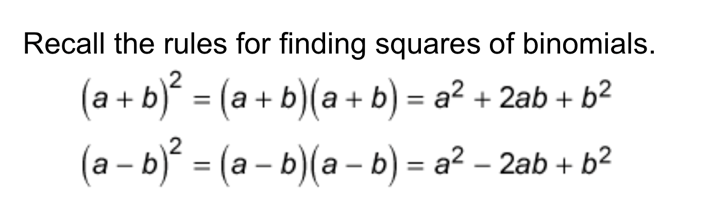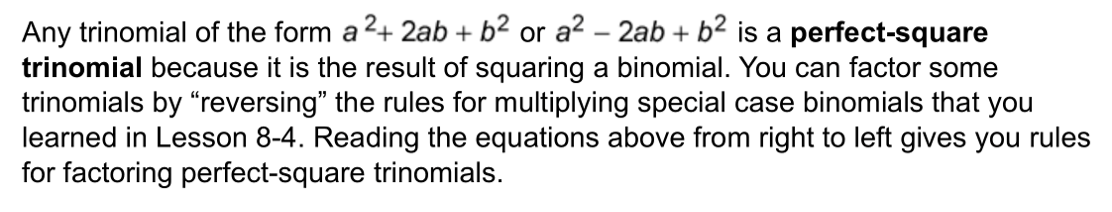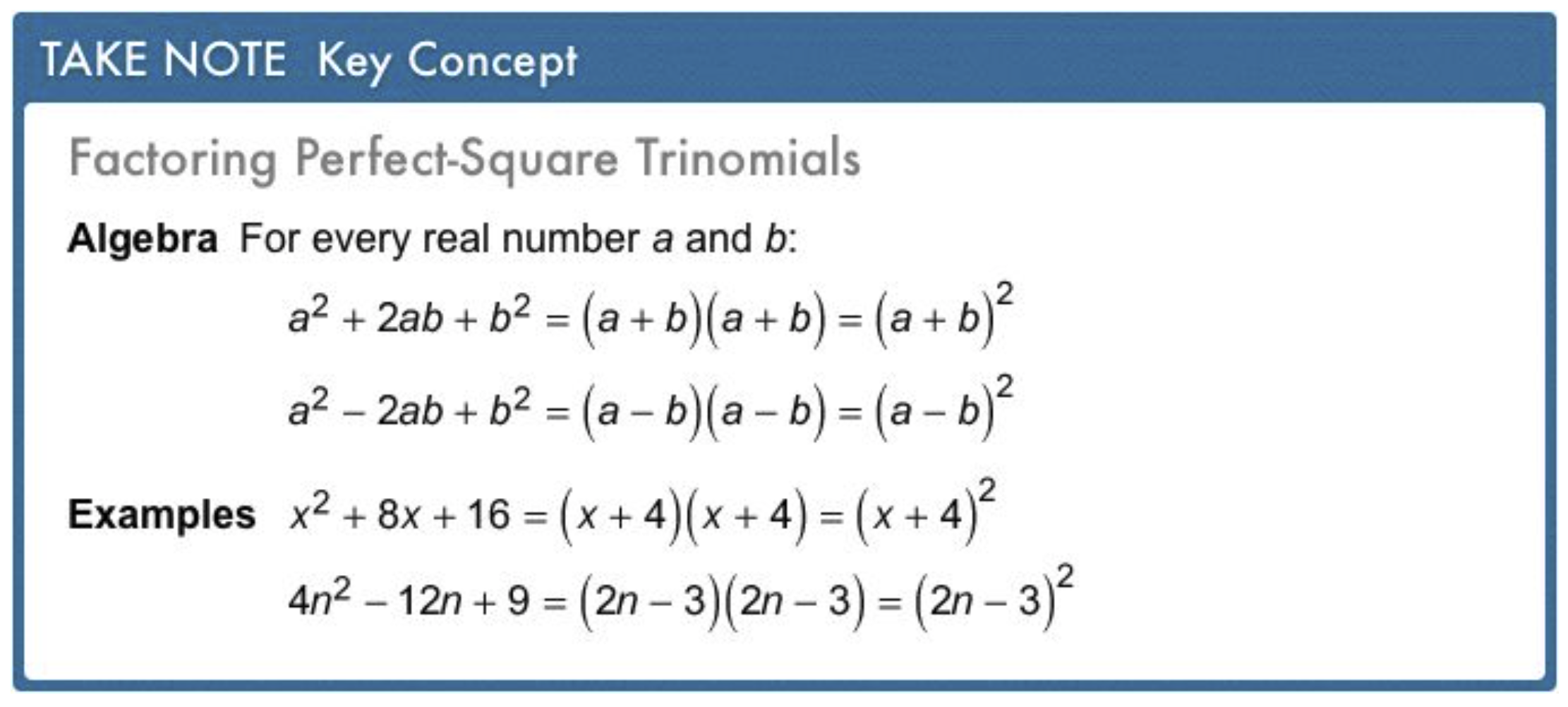2
2
10 pts
Problem 1 Got It?
A
B
C
D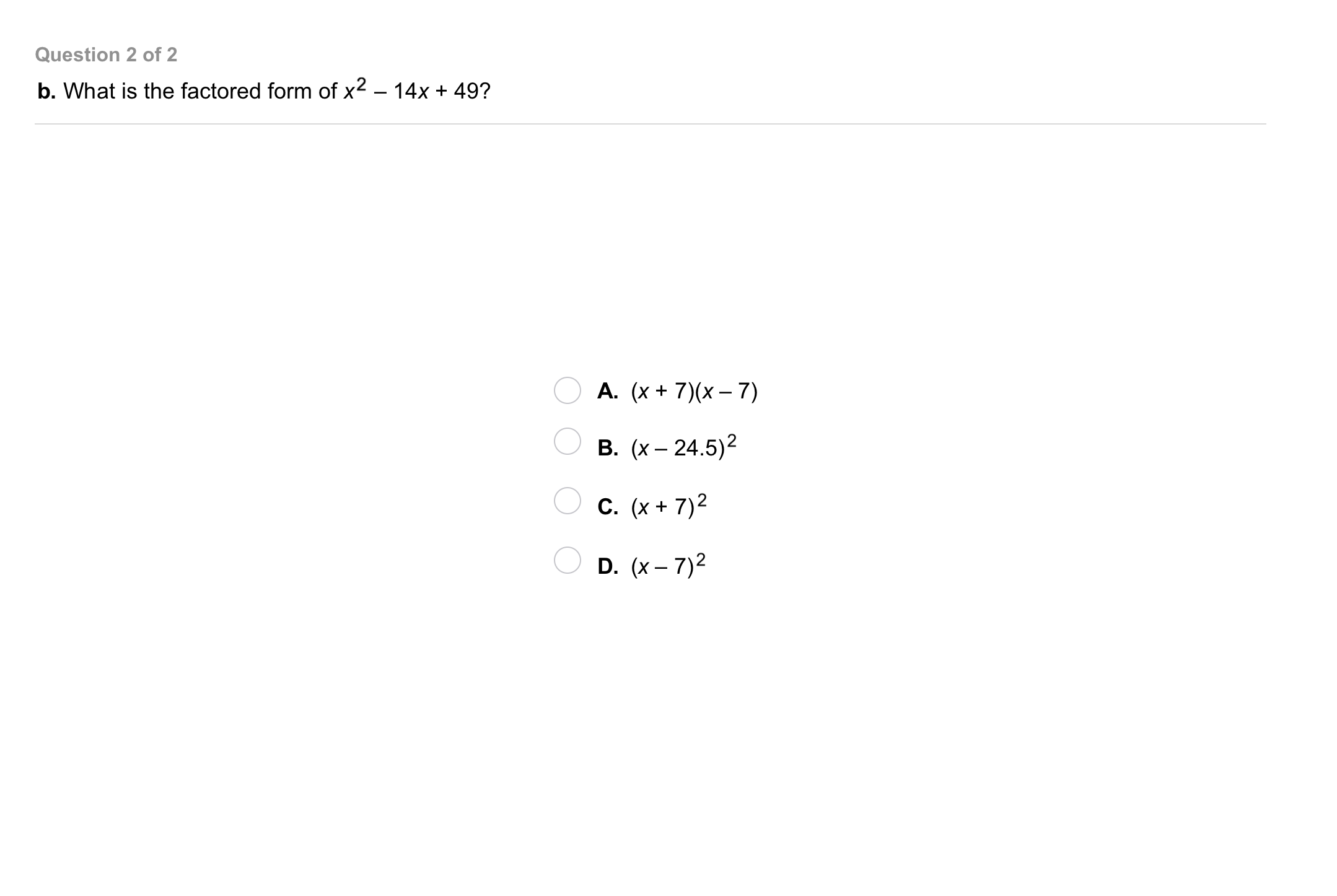3
3
10 pts
Problem 1 Got It?
A
B
C
D4
4
10 pts
Problem 2 Got It?
A
B
C
D5
10 pts
Vocabulary: Write a binomial that is a difference of squares.6
6
10 pts
Problem 3 Got It?
A
B
C
D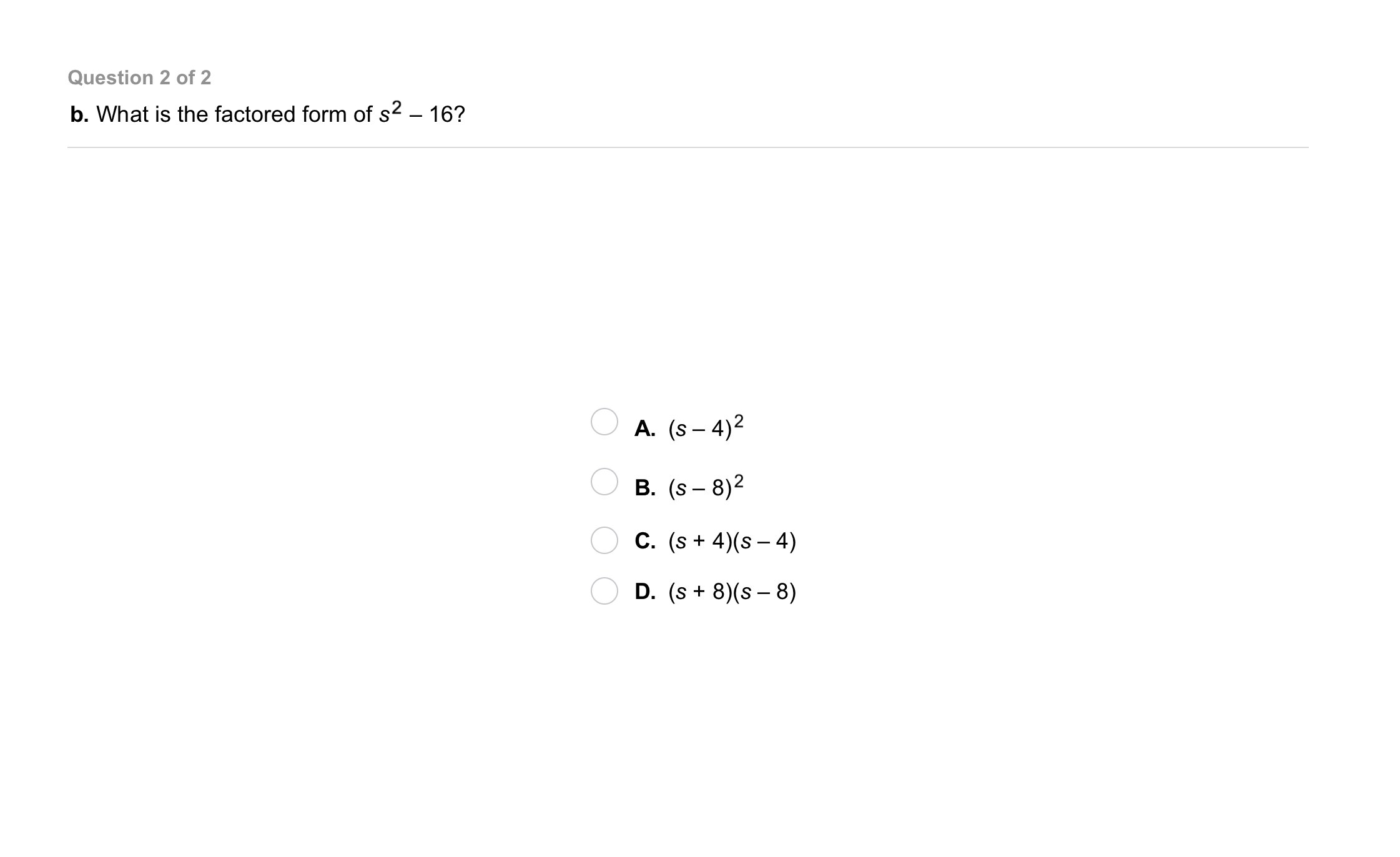7
7
10 pts
Problem 3 Got It?
A
B
C
D
8
10 pts
Problem 4 Got It? What is the factored form of the binomial?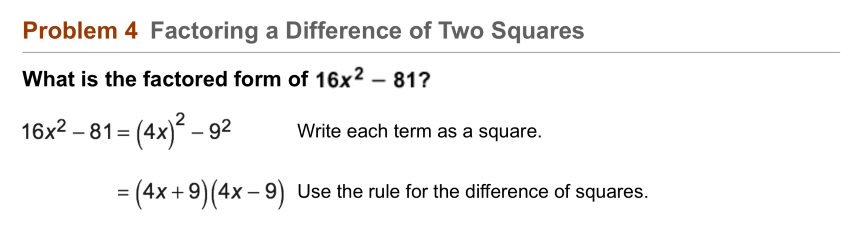9
9
10 pts
Problem 4 Got It? Reasoning: The expression below contains two perfect squares.
Can you use the method in Problem 4 to factor it? Explain your reasoning.10
10
10 pts
Problem 5 Got It?
A
B
C
D11
11
10 pts
Problem 5 Got It?
A
B
C
D12
12
10 pts
A
B
C
D13
13
10 pts
A
B
C
D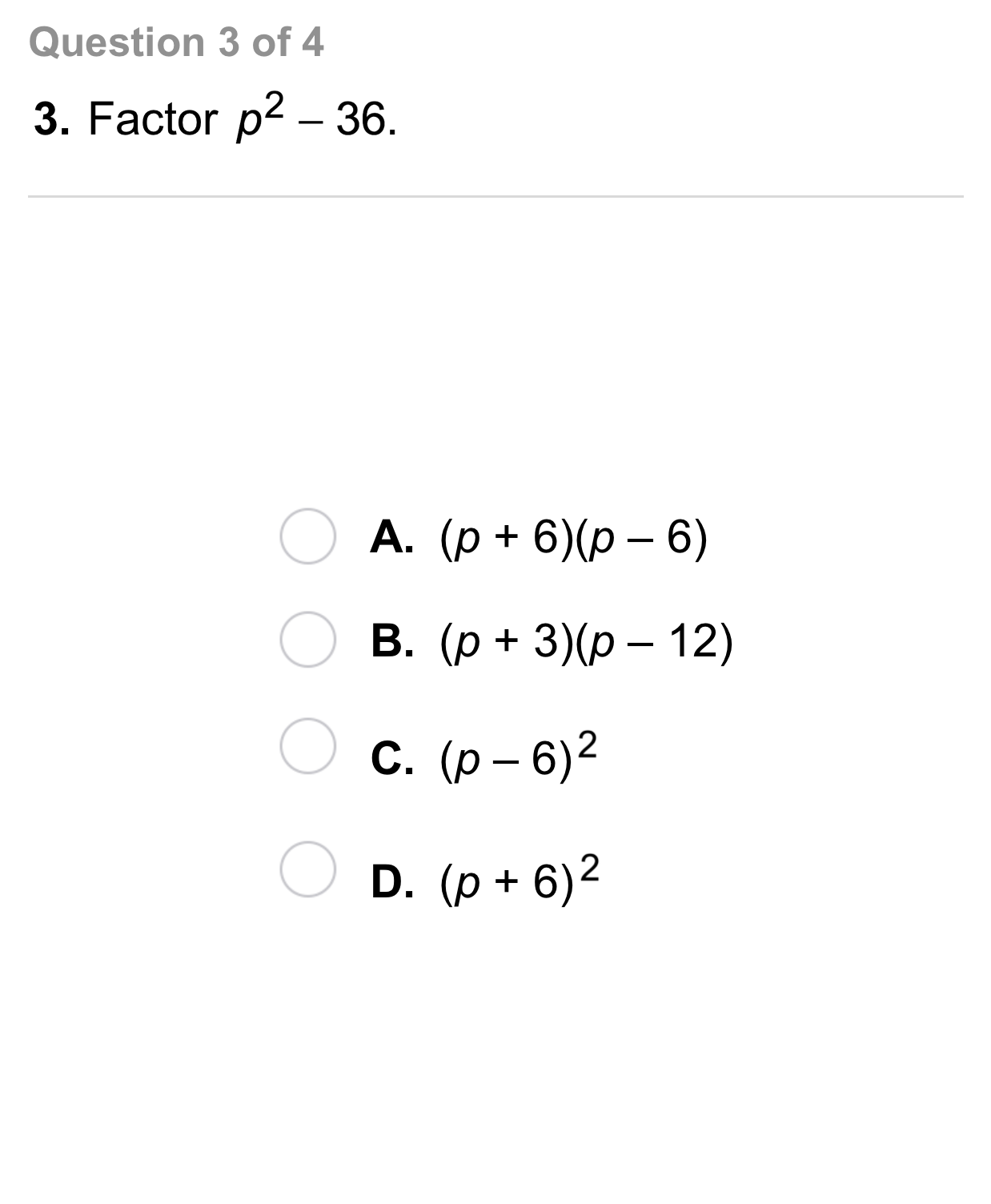14
14
10 pts
A
B
C
D15
15
10 pts
A
B
C
D16
5 pts
Reasoning: Identify the rule you would use to factor the expression.

Difference of two squares
Perfect-square trinomial
17
5 pts
Reasoning: Identify the rule you would use to factor the expression.

Perfect-square trinomial
Difference of two squares
18
5 pts
Reasoning: Identify the rule you would use to factor the expression.

Perfect-square trinomial
Difference of two squares20
10 pts
Review Lesson 8-6: Match the binomial factors with each trinomial to identify its factored form.
• (6a + 7)
• (4a + 3)
• (3a - 2)
• (2a + 3)
• (4a - 7)
• (3a - 5)
• 18a² + 9a - 14
• 8a² + 18a + 9
• 12a² - 41a + 35
21
10 pts
Review Lesson 8-2: Find the GCF of the terms of the polynomial.
Enter only a monomial.
22
10 pts
Review Lesson 8-2: Find the GCF of the terms of the polynomial.
Enter only a monomial.23
10 pts
Vocabulary Review: Identify the operators for each expression on the right.24
10 pts
Use Your Vocabulary: Match events in the left column with a corresponding special case of the event in the right column. Not all events will be used.
• a sport
• a meal
• a field trip
• a book
• an animal
• an assignment
• baseball
• a term paper
• a biography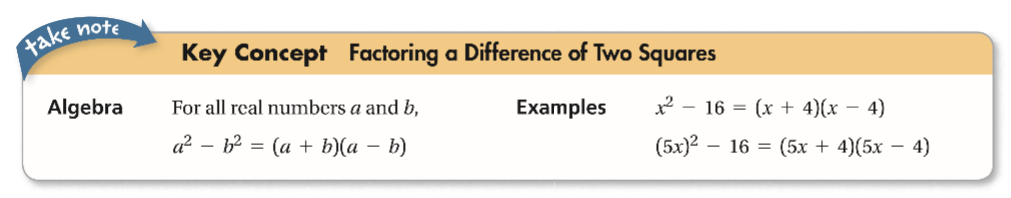25
100 pts
Notes: Take a clear picture or screenshot of your Cornell notes for this lesson. Upload it to the canvas. Zoom and pan as needed.

For a refresher on the Cornell note-taking system, click here.
26
10 pts
Reflection: Math Success
Add to my formatives list

Formative uses cookies to allow us to better understand how the site is used. By continuing to use this site, you consent to the Terms of Service and Privacy Policy.